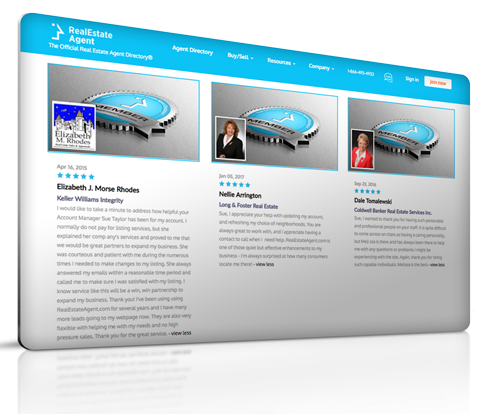# After-tax Cash Flow

## Definition of "After-tax cash flow"

Jennifer Dreggors, Real Estate Agent Berkshire Hathaway Executives

After-tax cash flow is a calculation method for companies’ financial performance to show their ability to generate cash flow through their operations. The after-tax cash flow formula works by adding costs that don’t include cash revenues (depreciation, restructuring costs, amortization, and impairments) to the company’s net income.

### What is After-Tax Cash Flow and How it Works?

Through after-tax cash flow, investors can understand the impact taxes have on their profits. This calculation method determines the company’s cash flow for undertaking an investment or project. Because depreciation is a non-cash expense while not being actual cash outflow, it is added to the net income. This is because depreciation acts as a tax shield, even if it is an expense. The same happens to amortization and other non-cash expenses.

#### The After-Tax Cash Flow Formula:

After-Tax Cash Flow = Net income + Depreciation + Amortization + Other Non-Cash Expenses.

So if we have a project with an operating income of \$1 million that has a depreciation value of \$90,000, and the company running the project pays a tax rate of 35\$, we get the net operating income through the following calculations:

• Firstly, we need to calculate the company’s earnings before taxes.

Earnings before taxes = Operating income - depreciated value

Earnings before taxes = \$1 million - \$90,000

Earnings before taxes = \$910,000

• Secondly, with earnings before taxes, we can calculate the net income.

Net Income = Earnings before taxes - (Tax Rate x Earnings before taxes)

Net Income = \$910,000 - (35% x \$910,000)

Net Income = \$910,000 - \$318,500

Net Income = \$591,500

• Finally, with the net income, we can use the after-tax cash flow formula to calculate.

After-Tax Cash Flow = Net Income + Depreciation + Other Non-Cash Expenses

After-Tax Cash Flow = \$591,500 + \$90,000

After-Tax Cash Flow = \$681,500

### Are you a realtor and want to become a member?

Residential Rental Commercial

#### Have a question or comment? We're here to help.